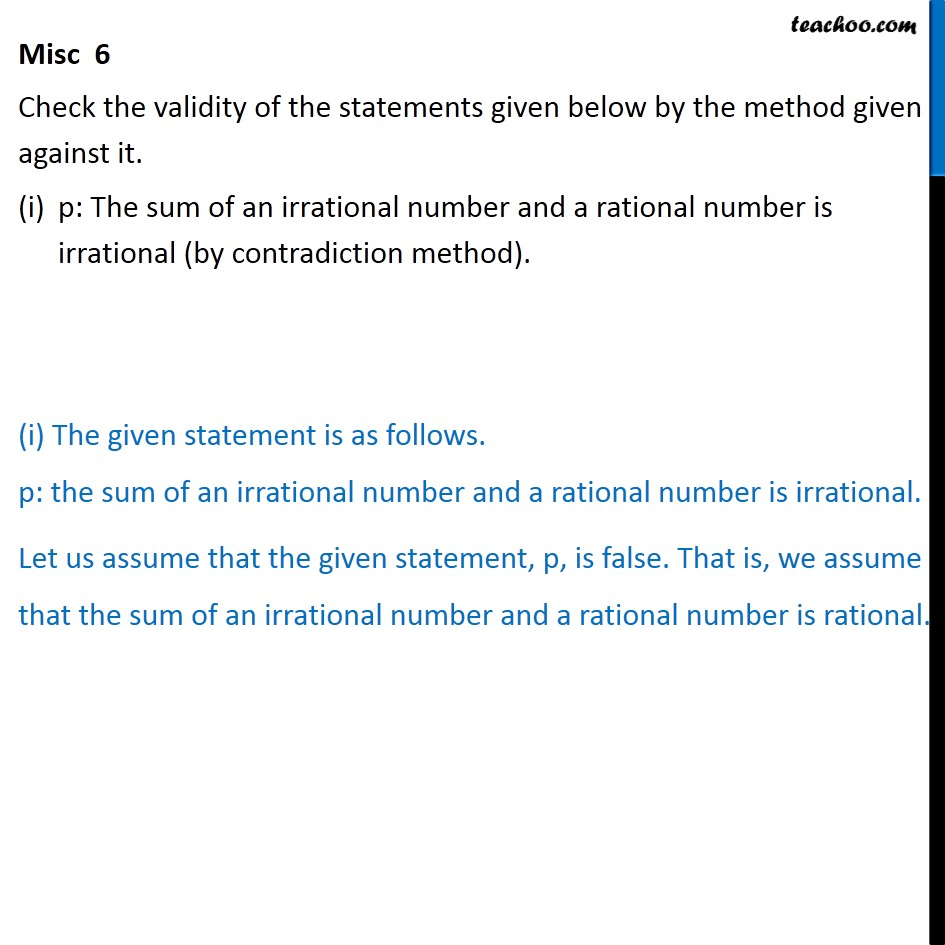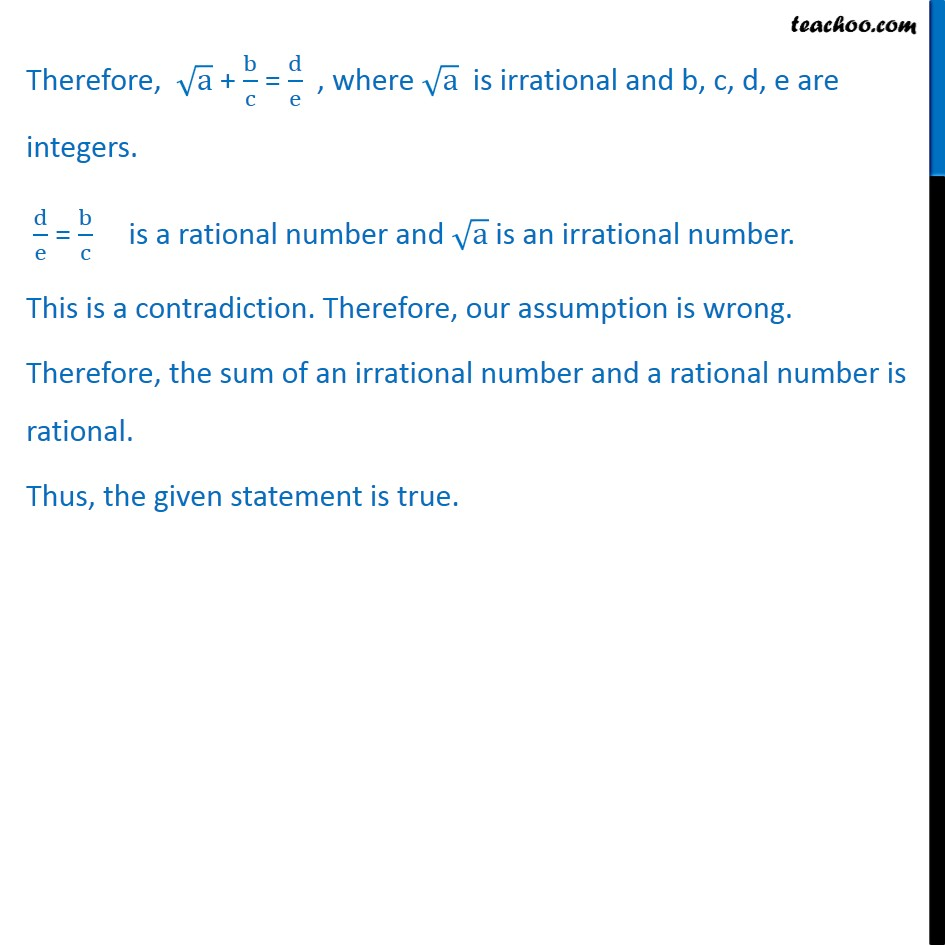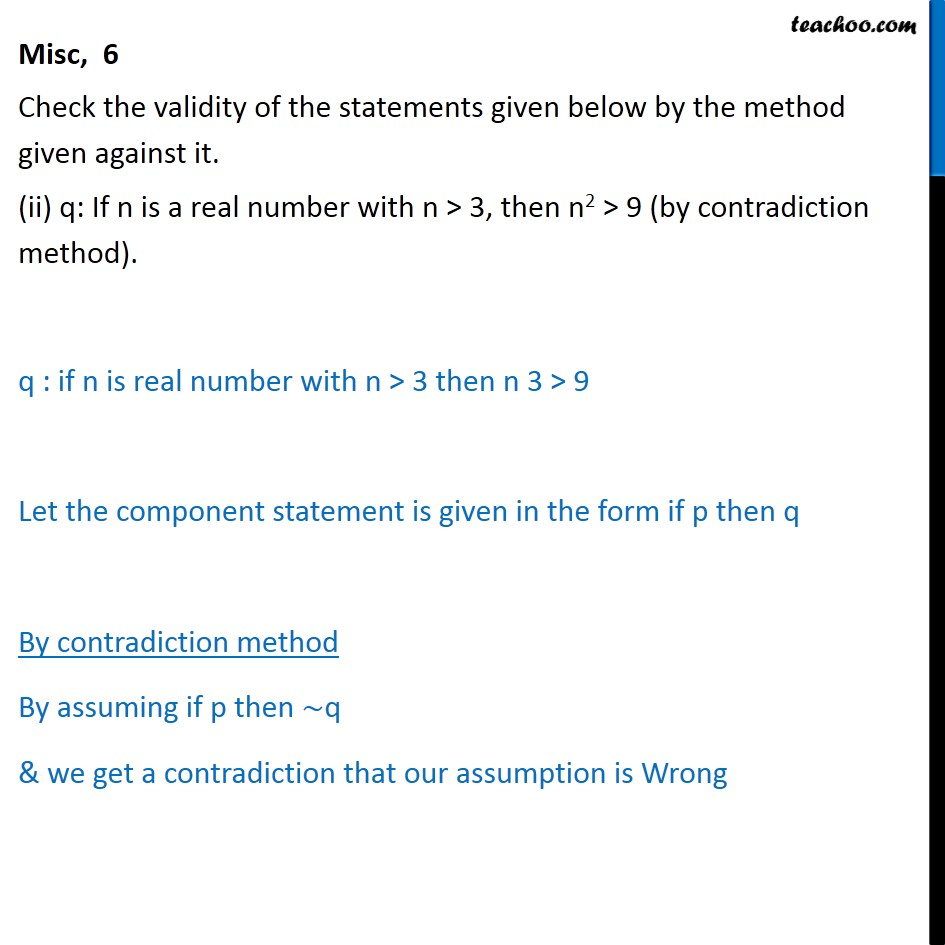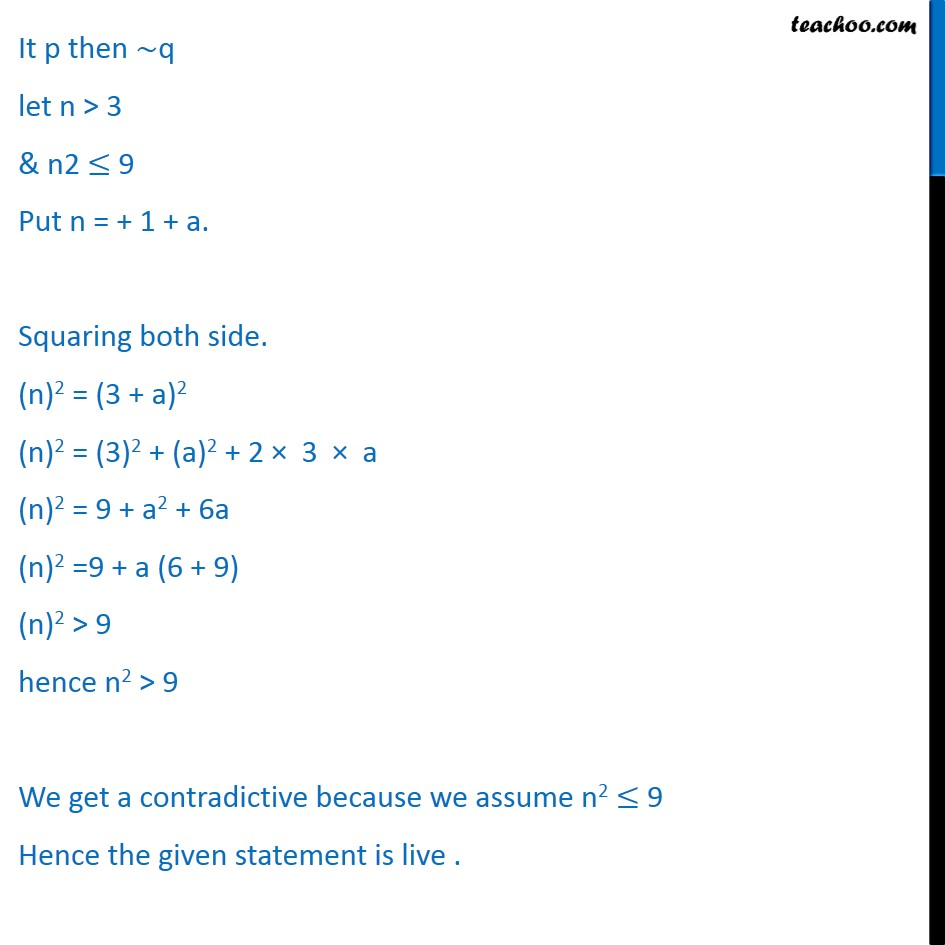Subscribe to our Youtube Channel - https://you.tube/teachoo

1. Chapter 14 Class 11 Mathematical Reasoning
2. Serial order wise
3. Miscellaneous

Transcript

Misc 6 Check the validity of the statements given below by the method given against it. p: The sum of an irrational number and a rational number is irrational (by contradiction method). (i) The given statement is as follows. p: the sum of an irrational number and a rational number is irrational. Let us assume that the given statement, p, is false. That is, we assume that the sum of an irrational number and a rational number is rational. Therefore, a + b c = d e , where a is irrational and b, c, d, e are integers. d e = b c is a rational number and a is an irrational number. This is a contradiction. Therefore, our assumption is wrong. Therefore, the sum of an irrational number and a rational number is rational. Thus, the given statement is true. Misc, 6 Check the validity of the statements given below by the method given against it. (ii) q: If n is a real number with n > 3, then n2 > 9 (by contradiction method). q : if n is real number with n > 3 then n 3 > 9 Let the component statement is given in the form if p then q By contradiction method By assuming if p then q & we get a contradiction that our assumption is Wrong It p then q let n > 3 & n2 9 Put n = + 1 + a. Squaring both side. (n)2 = (3 + a)2 (n)2 = (3)2 + (a)2 + 2 3 a (n)2 = 9 + a2 + 6a (n)2 =9 + a (6 + 9) (n)2 > 9 hence n2 > 9 We get a contradictive because we assume n2 9 Hence the given statement is live .

Miscellaneous